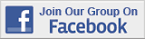How to Test for Equal and Unequal Variance (F Test)URL: https://www.youtube.com/watch?v=tscL1fzjSTYDESCRIPTION: Eugene O'Loughlin shows how to use an F test to decide whether to assume equal variances (7:22)CATEGORY: Excel TutorialsSUBFIELD: Political Science DataRSS LINK: NoVIDEO: Yes
 How to Test for Equal and Unequal Variance (F Test)URL: https://www.youtube.com/watch?v=tscL1fzjSTYDESCRIPTION: Eugene O'Loughlin shows how to use an F test to decide whether to assume equal variances (7:22)CATEGORY: Excel TutorialsSUBFIELD: Political Science DataRSS LINK: NoVIDEO: Yes
 How to Test for Equal and Unequal Variance (F Test)URL: https://www.youtube.com/watch?v=tscL1fzjSTYDESCRIPTION: Eugene O'Loughlin shows how to use an F test to decide whether to assume equal variances (7:22)CATEGORY: Excel TutorialsSUBFIELD: Political Science DataRSS LINK: NoVIDEO: Yes
 How to Test for Equal and Unequal Variance (F Test)URL: https://www.youtube.com/watch?v=tscL1fzjSTYDESCRIPTION: Eugene O'Loughlin shows how to use an F test to decide whether to assume equal variances (7:22)CATEGORY: Excel TutorialsSUBFIELD: Political Science DataRSS LINK: NoVIDEO: YesOver 18,000 Members!